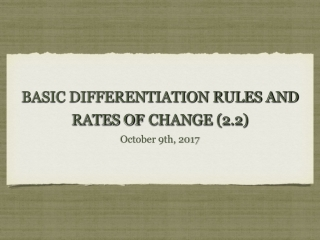DownloadDownload PresentationBASIC DIFFERENTIATION RULES AND RATES OF CHANGE (2.2)

# BASIC DIFFERENTIATION RULES AND RATES OF CHANGE (2.2)

Télécharger la présentation## BASIC DIFFERENTIATION RULES AND RATES OF CHANGE (2.2)

- - - - - - - - - - - - - - - - - - - - - - - - - - - E N D - - - - - - - - - - - - - - - - - - - - - - - - - - -
##### Presentation Transcript

1. October 9th, 2017 BASIC DIFFERENTIATION RULES AND RATES OFCHANGE (2.2)

2. I. THE CONSTANT RULE Thm. 2.2: The Constant Rule: The derivative of a constant function is 0. So, if c is a real number, then .

3. II. THE POWER RULE Thm. 2.3: The Power Rule: If n is a rational number, then the function is differentiable and . For f to be differentiable at x=0, n must be a number such that is defined on an interval containing 0. *When n=1, . Therefore, .

4. A. USING THE POWER RULE Ex. 1: Find the derivative of each function. (a) (b) (c) (d) (e) (f)

5. You Try: Find the derivative of each function. (a) (b) (c) (d)

6. B. FINDING THE SLOPE OF A GRAPH Ex. 2: Find the slope of the graph of when (a) x = -2 (b) x = 0 (c) x = 2

7. You Try: Find the slope of the graph of when (a) x = -1 (b) x = 1

8. C. FINDING AN EQUATION OF A TANGENT LINE Ex. 3: Find an equation of the tangent line to the graph of when x = 2.

9. You Try: Find an equation of the tangent line to the graph of when x = -3.

10. III. THE CONSTANT MULTIPLE RULE Thm. 2.4: The Constant Multiple Rule: If f is a differentiable function and c is a real number, then cf is also differentiable and .

11. Ex. 4: Find the derivative of each function. (a) (b) (c) (d)

12. IV. THE SUM AND DIFFERENCE RULES Thm. 2.5: The Sum and Difference Rules: The sum or difference of two differentiable functions f and g is also differentiable and

13. V. DERIVATIVES OF SINE AND COSINE Thm. 2.6: Derivatives of Sine and Cosine Functions:

14. VI. RATES OF CHANGE *The function s is called the position function and gives the position of an object with respect to the origin as a function of time t. The change in position over a period of time is given by and we know rate = distance/time, so we know the average velocity is average velocity = (change in distance)/(change in time) = .

15. AVERAGE VS INSTANTANEOUS * The average velocity (or average rate of change of a function) is found by the slope formula between two points on the position function (utilizes the secant line). *The instantaneous velocity (or instantaneous rate of change of a function) is found by the derivative at a single point (utilizes the tangent line.

16. A. FINDING AVERAGE VELOCITY OF A FALLING OBJECT Ex. 7: Given the position function , find the average velocity over the interval [2, 2.1].

17. You Try: Given the position function s(t)= sin t, find the average velocity over the interval [0, ].

18. *The instantaneous velocity of an object at time t is So, the velocity function v(t) is given by the derivative of the position function s(t). *The speed of an object is given by the absolute value of the velocity (velocity has direction, but speed cannot be negative).

19. *The position of a free-falling object (neglecting air resistance) under the influence of gravity is given by where is the initial height, is the initial velocity, and g is the acceleration due to gravity. (On Earth, feet per second per second or meters per second per second).

20. B. USING THE DERIVATIVE TO FIND VELOCITY Ex. 8: At time t = 0, a person jumps off a cliff that is 980 meters above the ground. The position of the person is given by where s is measured in meters and t is in seconds. (a) When does the person hit the ground? (b) What is the person’s velocity at impact?